# Solving cubish puzzles

2022-10-07: this is work in progress.

I love puzzles. Among them is a class of discrete puzzles that are not my favorites, but that are still entertaining.

They are discrete in the sense that they are made of small pieces, small cubes, assembled into big pieces, and the positions of the small cubes are very limited, fitting to some grid. (Some puzzles are not discrete, think about Tangram for example.)

The goal is to assemble the pieces so that you create a big shape. The simplest shape is a big cube as seen in the first puzzle below.

I love programming. Among the various programming activities available to the human brain is a class of tasks that consist of modelling some reality from the physical world and let the computer study that reality, in a way or another.

Mix both things and you will want to write a program to solve those puzzles.

For those cubish puzzles, the goal is obvious: find the positions of the various pieces to fill the big shape.

So let's do this! For it's... fun. Isn't it?

## First puzzle

We will solve several puzzles. Here is a picture of the first one, in its target position, a 3x3x3 big cube.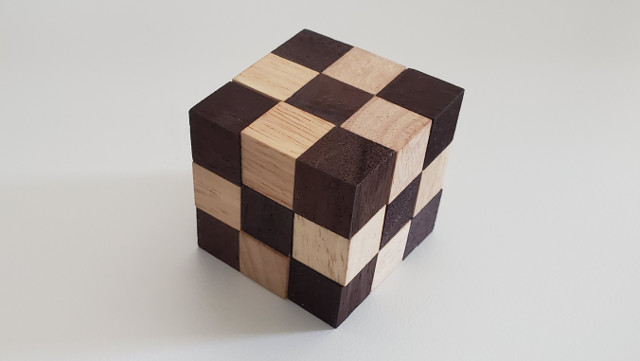And here is a picture of it in its initial position. Actually, it's just another position, but one that lets us see the various connections of the 27 small cubes. It's also kind of canonical in the sense that each small cube is either on the right or above the previous one, all of them put on the same plane.

Yes, we actually have two canonical positions, depending from which side you start. The choice is arbitrary. The small cube 26 could have been chosen as 0 instead. If you need to compare two puzzles you need to be careful to check both positions.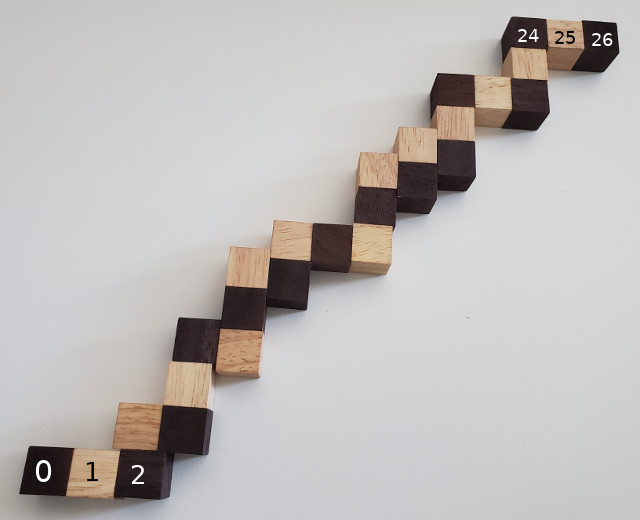I numbered the small cubes, from 0 to 26, because the solving algorithm processes them one by one, in the order given by their number.

Here comes a small video of me playing with this puzzle so that you understand how it moves. (I also start playing with the second puzzle at the end of the video. I was too lazy to cut that part.) (It's difficult to manipulate those puzzles with only one hand when the other holds a phone to record a video!) (The video is super compressed for size constraints on my small web server but I think it's enough to understand how the puzzle moves.)

The code to solve this first puzzle is cube_puzzle_0.tar.gz.

## The solving algorithm

The simplest is to explore all possible positions and print something on the screen when a solution has been found, that is when we assigned a position to all the small cubes.

To explore all positions, you put the small cube n in a valid position and you then process the following cubes. You try all valid positions. Once you put all the small cubes somewhere you have a solution.

The formulation of the algorithm is clearly recursive.

Note that we can also use a non-recursive code, because the recursion is very simple here. The transformation is left as an exercise to the reader. It's not hard. But not trivial either. You need to deal with the storage of x, y, and z seen in the recursive version and manipulate them when you start the processing of a new cube and when you go back to previous cube. Figure it out if you feel motivated, it's a funny exercise. The code will end up more verbose than the recursive version though.

There is no thinking in there, it tries all possible positions. It's a "brute force" approach. If there are a lot of positions it will take a lot of time. But in practice, it turns out that it's fast, so I won't look for better algorithms. What? Yes, I'm lazy. And a bit stupid too, yes. But that works!

Here is a sketch of the code to do that.

```void solve(int cube)
{
if (cube == 27) {
printf("solution\n");
return;
}
for (x = 0; x < 3; x++) for (y = 0; y < 3; y++) for (z = 0; z < 3; z++) {
if (check_constraints(cube, x, y, z))
solve(cube + 1);
}
}
```

In fact we don't necessarily need to process the small cubes in the chosen order. It just seemed convenient when that decision was taken.

## The representation of the cubes

How to put in a computer program the small cubes and their connections to each other?

Looking at the initial position, we could think of using (x,y) coordinates. We could put the little cube 0 at (0,0), the little cube 1 at (1,0) and so on and store those positions in an array. But then what? What would the function check_constraints() do with that?

So instead, we will simply store the various constraints, for all the small cubes. We will store them in an array, from cube 0 to cube 26, in order, for it's this order that was chosen to process the small cubes.

What are those contraints?

Well, for cube 0 it has no constraint and can be put anywhere in the 3x3x3 big cube.

Cube 1 has to be near cube 0. And that's it. No other constraint.

Cube 2 has to be near cube 1 and cubes 0, 1, and 2 have to be aligned. And no other constraint.

Cube 3 has to be near cube 2 and this time cubes 1, 2, and 3 need to be not aligned.

And so on for all the following cubes.

So the only constraints we seem to need are "near", "aligned" and "not aligned". Is it enough? How to prove it?

It seems to be enough yes. And I can't prove it. Just my program uses only those constraints and gives some results that look correct. This is not a real mathematical proof, it's indirect, but that does the job as far as I am concerned. It is a bit lame, I agree. But I have zero idea on how to prove in a mathematical way that it's indeed enough. Shame on me? Yes.

Here is the code for those constraints for the first puzzle. "Near" is represented as TOUCH, not NEAR.

```int *puzzle_1 = (int []){
CUBE, 0,   /* no constraint */
CUBE, 1,   TOUCH, 0,
CUBE, 2,   TOUCH, 1, ALIGNED, 0, 1,
CUBE, 3,   TOUCH, 2, NOT_ALIGNED, 1, 2,
CUBE, 4,   TOUCH, 3, NOT_ALIGNED, 2, 3,
CUBE, 5,   TOUCH, 4, NOT_ALIGNED, 3, 4,
CUBE, 6,   TOUCH, 5, ALIGNED, 4, 5,
CUBE, 7,   TOUCH, 6, NOT_ALIGNED, 5, 6,
CUBE, 8,   TOUCH, 7, NOT_ALIGNED, 6, 7,
CUBE, 9,   TOUCH, 8, ALIGNED, 7, 8,
CUBE, 10,  TOUCH, 9, NOT_ALIGNED, 8, 9,
CUBE, 11,  TOUCH, 10, NOT_ALIGNED, 9, 10,
CUBE, 12,  TOUCH, 11, NOT_ALIGNED, 10, 11,
CUBE, 13,  TOUCH, 12, ALIGNED, 11, 12,
CUBE, 14,  TOUCH, 13, NOT_ALIGNED, 12, 13,
CUBE, 15,  TOUCH, 14, ALIGNED, 13, 14,
CUBE, 16,  TOUCH, 15, NOT_ALIGNED, 14, 15,
CUBE, 17,  TOUCH, 16, NOT_ALIGNED, 15, 16,
CUBE, 18,  TOUCH, 17, NOT_ALIGNED, 16, 17,
CUBE, 19,  TOUCH, 18, NOT_ALIGNED, 17, 18,
CUBE, 20,  TOUCH, 19, ALIGNED, 18, 19,
CUBE, 21,  TOUCH, 20, NOT_ALIGNED, 19, 20,
CUBE, 22,  TOUCH, 21, ALIGNED, 20, 21,
CUBE, 23,  TOUCH, 22, NOT_ALIGNED, 21, 22,
CUBE, 24,  TOUCH, 23, ALIGNED, 22, 23,
CUBE, 25,  TOUCH, 24, NOT_ALIGNED, 23, 24,
CUBE, 26,  TOUCH, 25, ALIGNED, 24, 25,
-1
};
```

When checking contraints when processing a cube n, we only need to check the constraints of that cube and those already put in position, that is the previous cubes in our algorithm. And since the previous cubes don't move when we put a new one, their constraints will still be respected and we don't need to check them again. So we only need to check the constraints for the cube n.

Here again, this is not very mathematical, it's more intuitive thinking, but again, the code seems to work.

I think it's a good thing that I don't write software for critical applications (transport, health, space, ...).

I chose a flat array for the constraints. It's arbitrary. But I then need some kind of "tag" to know when to start and stop processing contraints for a cube. This is the CUBE thing.

How do we check the contraints? Here is the code.

```int *check_constraints(int cube, int x, int y, int z, int *puzzle, pos_t *p)
{
int c1, c2;
int delta;
int delta_x1, delta_x2;
int delta_y1, delta_y2;
int delta_z1, delta_z2;
int aligned;

while (*puzzle != CUBE && *puzzle != -1) {
switch (*puzzle) {
case TOUCH:
c1 = puzzle;
delta = abs(p[cube].x - p[c1].x)
+ abs(p[cube].y - p[c1].y)
+ abs(p[cube].z - p[c1].z);
if (delta != 1) return NULL;
puzzle += 2;
break;
case ALIGNED:
case NOT_ALIGNED:
c1 = puzzle;
c2 = puzzle;
delta_x1 = p[c2].x - p[c1].x;
delta_x2 = p[cube].x - p[c2].x;
delta_y1 = p[c2].y - p[c1].y;
delta_y2 = p[cube].y - p[c2].y;
delta_z1 = p[c2].z - p[c1].z;
delta_z2 = p[cube].z - p[c2].z;
aligned = delta_x1 == delta_x2
&& delta_y1 == delta_y2
&& delta_z1 == delta_z2;
if (*puzzle == ALIGNED && !aligned) return NULL;
if (*puzzle == NOT_ALIGNED && aligned) return NULL;
puzzle += 3;
break;
}
}
return puzzle;
}
```

TOUCH means that the two touching cubes have a distance of 1. We can cheat a bit and not really compute the distance. Only the delta of each x, y, and z coordinates are computed and if the sum is 1, we're good. (Mathematical proof? you need one? really?)

For aligned/not aligned we cheat again. We only deal with cubes following each other. So they are aligned if the deltas for their x, y, and z coordinates for the first two cubes are equal to the deltas for the last two cubes. And they are not aligned if the test for "aligned" is false. (Math proof, where are you? not in my brain as it seems.)

Now, read the code for all the other gory details. The solve() function is more complicated than what is given above. It has to deal with "busy", that is: you don't put two cubes at the same position. And it also has to deal with states, especially when printing a solution we need the coordinates of all the small cubes.

## Too many solutions

With all this, what does the program do? Let's see.

```/tmp/puzzle> cd cube_puzzle_0
/tmp/puzzle/cube_puzzle_0> make
gcc -g -Wall -c -o main.o main.c
gcc -g -Wall -c -o puzzle.o puzzle.c
gcc -g -Wall -o solve main.o puzzle.o
/tmp/puzzle/cube_puzzle_0> ./solve |grep solution > solution_1.txt
/tmp/puzzle/cube_puzzle_0> wc -l solution_1.txt
48 solution_1.txt
```

What? 48 solutions? How come? I would expect only one.

Ah, but wait. It's all about rotation of the big cube. We look for all solutions without taking into account rotations. The big cube has six faces. Imagine a solution. Now, turn the big cube so that the face facing you changes. For my program it's another solution, but for us humans it's the same.

So we need to get rid of rotations.

But how many rotations do we have?

Six faces.

Imagine face A faces you. You turn the big cube by 1/4 turn, still face A facing you. You get another position. You can do it again and again. In the end you will have each one of the four touching faces of face A pointing up. So that's four rotations when face A faces you.

Now face B faces you. Again, four rotations.

Same for faces C, D, E and F.

In the end we have six times four rotations, that is 24.

So we divide all the solutions we have by 24. Remains 2. What? Two solutions? How come? It should be unique! (okay, it should be 24, taking into account the rotations, unless I messed up somewhere in my thinking)

## Canonical position

To get rid of the rotations problem, once we have a solution, we can look for a canonical position for it, that can be reached by rotating the cube. What can this canonical thing be? We can force some small cubes to some given positions in the big cube. We have to choose the small cubes so that once put at the forced positions there is only one possibility for all the other small cubes.

I claim that choosing 3 small cubes that are touching and not aligned is enough. We say that the first small cube has to be at (0,0,0), the next at (0,0,1) and the third at (0,1,1) and we're good. (Proof that it's enough? What proof? I said "I claim"! So this is proved! Done!)

And when the program finds a solution, it has to call a new function normalize() that will rotate and translate all the small cubes so that the chosen small cubes are at the right position.

But if we do only that, we may have some small cubes with coordinates out of [0, 1, 2], so we need a final translation to put everything back into [0, 1, 2] for the three coordinates. (In the code, the function normalize() does this translation by first looking for the minimum value of x, y, and z, and then substracting it to all the coordinates, leading to them being in [0, 1, 2]. What? You want a proof for that too?)

I could have solved a system of equations to find, say, a matrix to do the transform but I'm super bad with matrices so instead I do things piece by piece. First one translation to put the first small cube at (0,0,0). Then three rotations to put the second small cube at (0,0,1). We first rotate around z, then x and then y (arbitrary choice). And yes it works. And no, still no proof!

For the third small cube to go to (0,1,1), we know that after the previous translation and rotations, it can only be at positions (1,0,1) or (0,1,1) or (-1,0,1) or (0,-1,1) (its z coordinate is good, only x and y have to be fixed) (picture it in your head if you can) (I should put some pictures here, too much words). I was too lazy to write code for each case, so this time I decided to solve some equations to get the rotation. If we note the rotation as the complex number a+ib we find out that a=y and b=x where the third small cube has coordinates (x,y,z). (Yes, we know that a rotation is a multiplication by a complex number.) The derivation to reach a=y and b=x can be seen in the source code. I'm not sure it's super clear though... But it works!

And the code is at cube_puzzle_1.tar.gz.

Let's see what we get now. Since we have 48 solutions, that should be two with the canonical position. (I secretely hope it's one and I messed up somewhere in my thinking about the number of rotations.)

```/tmp/puzzle> cd cube_puzzle_1
/tmp/puzzle/cube_puzzle_1> make
gcc -g -Wall -c -o main.o main.c
gcc -g -Wall -c -o puzzle.o puzzle.c
gcc -g -Wall -o solve main.o puzzle.o
/tmp/puzzle/cube_puzzle_1> ./solve |grep solution|sort|uniq > solution_1_canonical.txt
/tmp/puzzle/cube_puzzle_1> wc -l solution_1_canonical.txt
2 solution_1_canonical.txt
```

Ah, it's two. Okay, so be it. Is it really two? Hum, looking at the solutions, yes, they are different. We can see that the x of all the cubes are reversed, that is 0 becomes 2 and 2 becomes 0 (and 1 remains 1). Strange.

But let's move on to another puzzle! We'll see later about those two solutions. Maybe I'll write some visualization tool at some point to understand what happens here.

## Second puzzle

Here is a picture of the initial position of the second puzzle. The goal is the same as puzzle 1, so we don't show it.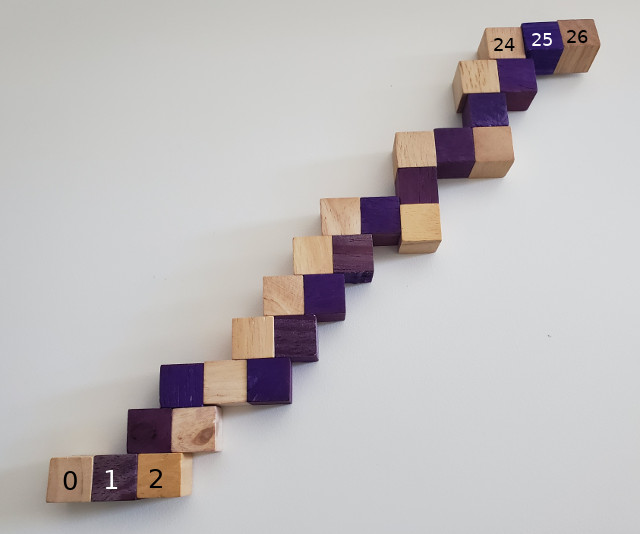Let me show you both puzzles side by side so you can see they are different (which was a big surprise to me to be honest).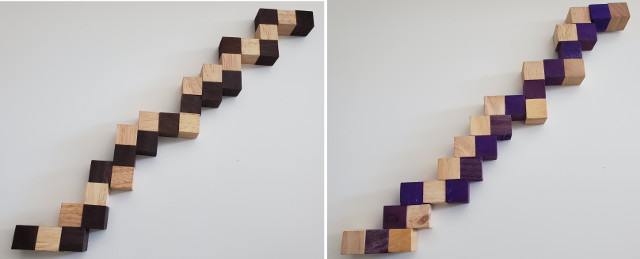Here is the code: cube_puzzle_2.tar.gz

The code for this second puzzle is the same as for the first puzzle. I just added the constraints for the second puzzle. So I won't talk about this code more than that.

And what do we get?

```/tmp/puzzle> cd cube_puzzle_2
/tmp/puzzle/cube_puzzle_2> make
gcc -g -Wall -c -o main.o main.c
gcc -g -Wall -c -o puzzle.o puzzle.c
gcc -g -Wall -o solve main.o puzzle.o
/tmp/puzzle/cube_puzzle_2> ./solve |grep solution|sort|uniq > solution_2_canonical.txt
/tmp/puzzle/cube_puzzle_2> wc -l solution_2_canonical.txt
6 solution_2_canonical.txt
```

What? Six solutions now... This puzzle is even worse.

Now the question is: can we design a similar puzzle with only one solution? Exercise for the reader, I'm way too lazy for this question.

## Third puzzle

Let's add more diversity to our zoo. The third puzzle is different. The goal is the same but the small cubes are organized differently.

The target position is as follows.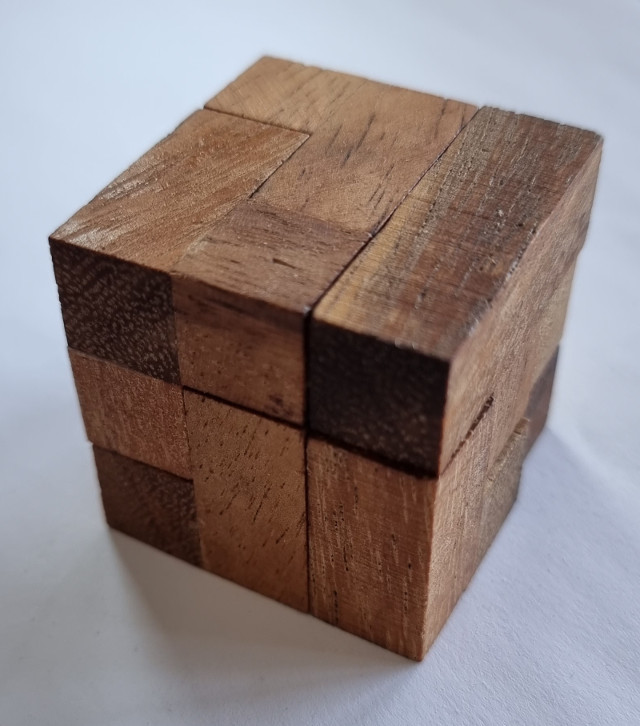Here are all the pieces of the puzzle, numbered from 1 to 7.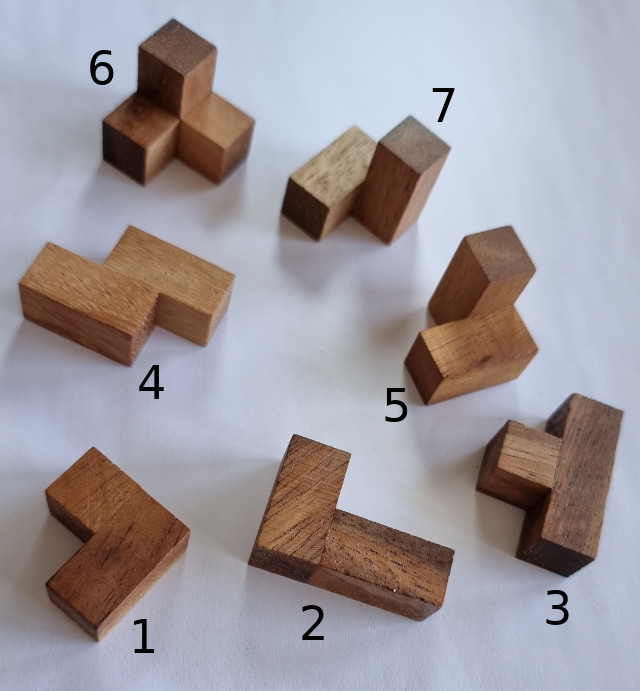For this puzzle, we need more constraints to describe the pieces which are more rigid than before. In the previous puzzles there were holes on the small cubes and some kind of string inside, like a necklace, but with some tension to keep things tight.

Now the cubes are either glued to each other or completely disconnected. We can still use TOUCH and ALIGNED/NOT_ALIGNED but we need some more to force the position of some small cubes.

For a small cube, TOUCH defines 6 positions with respect to the previous small cube (which has six faces).

ALIGNED defines 1 position with respect to the two previous small cubes.

NOT_ALIGNED defines 4 positions.

Now look at piece 7.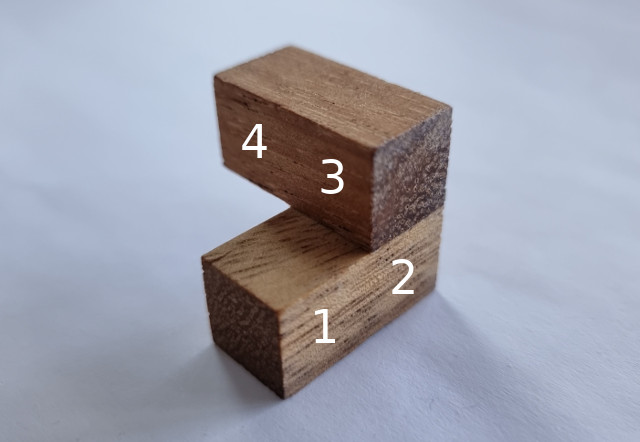With only TOUCH and NOT_ALIGNED there is ambiguity for the small cube 4. 4 touches 3. 2, 3, and 4 are not aligned. But with only those constraints there are four possible positions. 4 is always touching 3. Then it can be on top of 1. Or it can be touching 3 as it does on the picture, on the left. Or it can touch it on the right (opposite position). Or even be at the end of 3, like piece 4 from the previous picture.

If we introduce LEFT then we can say that 4 has to be on the LEFT of (1,2,3), and then there is only one possibility.

LEFT is also enough to describe pieces 5 and 6.

For piece 4 we can introduce say VEC, that would mean that the vector (1,2) is equal to the vector (3,4) (I did not number the small cubes of piece 4, but it would be like piece 7 with its small cube 4 at the right position to look like piece 4, say small cubes 1, 2, 3, and 4 are in the same plane) (I hope it's clear).

And that should be enough to describe the 7 pieces.

Another problem now pops up.

Some pieces can occupy a given position in the 3x3x3 big cube in different ways, different rotations. If we don't eliminate all but one position we lose canonicity and we will have way too much solutions. Those solutions won't be eliminated by the normalize() function which works on the big 3x3x3 cube, not individual small cubes.

So we need a canonical position for individual pieces. What can it be? We can define an absolute order for the small cubes of a piece and eliminate all rotations that don't respect this order. We need to define some kind of measure. If we define x*9+y*3+z as this measure for a given small cube then we can introduce the ORDER constraint that is satisfied when the small cubes it uses are ordered with respect to the defined measure.

Proof that it works?

Okay, yes, proof is needed. For the pieces 1, 3, 4, 5, 6, and 7 (piece 2 has no symmetry) we will write a program that puts them in all possible positions in the 3x3x3 cube without the ORDER constraint. We will store all those positions. Then we do the same with the ORDER constraint this time and we check if we generate the same positions. But here a position is just bits set to 0 or 1 in the big 3x3x3 cube without caring of which small cube occupies which place where.

The code to check is check_order.tar.gz.

And... surprise! It works. Look.

```/tmp/puzzle> tar xf check_order.tar.gz
/tmp/puzzle> cd check_order
/tmp/puzzle/check_order> make
gcc -g -Wall -c -o main.o main.c
gcc -g -Wall -c -o puzzle.o puzzle.c
gcc -g -Wall -o solve main.o puzzle.o
/tmp/puzzle/check_order> ./solve |grep "solution full piece 1"|sort|uniq|wc -l
144
/tmp/puzzle/check_order> ./solve |grep "solution constrained piece 1"|sort|uniq|wc -l
144
/tmp/puzzle/check_order> ./solve |grep "solution full piece 3"|sort|uniq|wc -l
72
/tmp/puzzle/check_order> ./solve |grep "solution constrained piece 3"|sort|uniq|wc -l
72
/tmp/puzzle/check_order> ./solve |grep "solution full piece 4"|sort|uniq|wc -l
72
/tmp/puzzle/check_order> ./solve |grep "solution constrained piece 4"|sort|uniq|wc -l
72
/tmp/puzzle/check_order> ./solve |grep "solution full piece 5"|sort|uniq|wc -l
96
/tmp/puzzle/check_order> ./solve |grep "solution constrained piece 5"|sort|uniq|wc -l
96
/tmp/puzzle/check_order> ./solve |grep "solution full piece 6"|sort|uniq|wc -l
64
/tmp/puzzle/check_order> ./solve |grep "solution constrained piece 6"|sort|uniq|wc -l
64
/tmp/puzzle/check_order> ./solve |grep "solution full piece 7"|sort|uniq|wc -l
96
/tmp/puzzle/check_order> ./solve |grep "solution constrained piece 7"|sort|uniq|wc -l
96
```

Good. Good? Is the code correct? Oh my, that's a difficult question. If you look at the code there is a commented part in main.c that forces one cube to be in a corner of the big cube. Then it's easier to count solutions and do a manual check. I did that. It seems to be okay. That's the only proof I'm willing to give. (For the moment.)

Now let's glue all together and solve this puzzle. How many solutions do you think we get (removing symmetries for pieces and for the big cube). Only one? Hum? I bet on many many, there is too much freedom in there.

The code is cube_puzzle_3.tar.gz.

```/tmp/puzzle> tar xf cube_puzzle_3.tar.gz
/tmp/puzzle> cd cube_puzzle_3
/tmp/puzzle/cube_puzzle_3> make
gcc -g -Wall -c -o main.o main.c
gcc -g -Wall -c -o puzzle.o puzzle.c
gcc -g -Wall -o solve main.o puzzle.o
/tmp/puzzle/cube_puzzle_3> time ./solve |grep solution > solution_3_bad.txt
real    43m30.937s
user    44m2.931s
sys     3m13.286s
6767
```

And we have a problem... If we accept that there are 11520 solutions, with cube symmetries we should get 11520/24 = 480 unique solutions, not 6767.

Also it's very slow. Man! 43 minutes!

Time to dig for the bug... See you later.

## After a night of sleep

I got it! I think.

There is no bug in the code.

The problem is that the ORDER constraint and the function normalize() don't work well together, not eliminating all the 24 rotations for a solution.

Yesterday evening I went to bed with this bug in mind. This morning I woke up, listened to the radio for a few minutes and thought about it again. And the reason came, out of nowhere, with no thinking at all. I found the problem as by magic while sleeping.

This is not the first time it happens and is always a great mistery.

Bah, so be it.

So, how can I explain the issue?

A solution can exist in 24 rotations of the 3x3x3 big cube. The function normalize() will put 3 given small cubes to a specific position so that all the 24 rotations end up given the exact same solution for puzzles 1 and 2.

But for puzzle 3, a piece can be in more than one position for a given solution. So I introduced the ORDER constraint.

This constraint is not compatible with normalize().

Imagine that for a rotation of a solution the piece 7 is at some position. For another rotation of the same solution, the piece 7 is at the rotated position. Except that because of ORDER the small cubes of the piece 7 will be in their own canonical position, which may be the opposite of their position for the first rotation. So that after we call normalize() the piece 7 will not be in its canonical position, and the textual representation of the rotated solution will not be the same as the one of the first rotation.

That behavior exists with all the other pieces using the ORDER constraint.

How to solve this?

Hum, the simplest is to output a solution in a different format, like the one used by check_order so that how each individual piece is rotated does not matter.

Let's try that!

```/tmp/puzzle> gcc -Wall -o puzzle3_reformat_solution puzzle3_reformat_solution.c
/tmp/puzzle> cat cube_puzzle_3/solution_3_bad.txt | ./puzzle3_reformat_solution|sort|uniq|wc -l
960
```

And we have a problem... 11520 / 24 = 480, not 960. We have twice too many solutions. What is going on now?

Time to think!

But first let's remove the ORDER constraint and see what we get.

```/tmp/puzzle/cube_puzzle_3> make clean
rm -f solve *.o
/tmp/puzzle/cube_puzzle_3> make
gcc -g -Wall -O3 -c -o main.o main.c
gcc -g -Wall -O3 -c -o puzzle.o puzzle.c
gcc -g -Wall -O3 -o solve main.o puzzle.o
/tmp/puzzle/cube_puzzle_3> time ./solve |\grep -i solution > solution_3_bad.no_order.txt

real    434m45.642s
user    448m50.737s
sys     31m15.972s
-rw-r--r-- 1 tmp umts 654704640 Jun 25 05:30 solution_3_bad.no_order.txt
-rw-r--r-- 1 tmp umts 625M Jun 25 05:30 solution_3_bad.no_order.txt
/tmp/puzzle/cube_puzzle_3> grep solution solution_3_bad.no_order.txt |wc -l
1105920
/tmp/puzzle/cube_puzzle_3> grep solution solution_3_bad.no_order.txt |sort|uniq|wc -l
46080
```

Oh, it takes 430 minutes, that's a lot.

We get 1105920 solutions, this is what we want. This is 11520*3*2*2*2*2*2. 11520 is the number of solutions when using ORDER. The 3*2*2*2*2*2 is the number of inner rotations for the small pieces. Piece 6 has 3. Pieces 1, 3, 4, 5, and 7 have 2. Piece 2 has none (well, only 1 to be correct).

## After one or two hours of thinking

Just before going to bed, I thought a bit. And I got it! It's that normalize() uses piece 1 to rotate a solution to reach the canonical position.

But before the rotation happens, piece 1 may be in 2 positions in the non-canonical solution. ORDER forces only 1. But it may not be always the same for the 24 rotations of a solution. So after the rotation we will have 2 final positions depending on the position of piece 1. Thus, in the end, 960 solutions instead of the expected 480.

How to solve? Simple. Use small cubes of piece 2 in normalize() because piece 2 has only 1 possible position in a solution.

We are lucky to have piece 2. What to do if all the pieces have 2 or more possible positions? Hum, I don't know. And I don't need to know. So I won't dig further.

Yes, my brain is lazy.

Let's see what we get.

```/tmp/puzzle> cd cube_puzzle_3
/tmp/puzzle/cube_puzzle_3> patch < ../cube_puzzle_3_patch.txt
patching file puzzle.c
/tmp/puzzle/cube_puzzle_3> make
gcc -g -Wall -c -o main.o main.c
gcc -g -Wall -c -o puzzle.o puzzle.c
gcc -g -Wall -o solve main.o puzzle.o
/tmp/puzzle/cube_puzzle_3> time ./solve |grep -i solution > solution_3_good.txt

real    43m38.581s
user    44m55.739s
sys     2m57.829s
/tmp/puzzle/cube_puzzle_3> ls -l solution_3_good.txt
-rw-r--r-- 1 tmp umts 6819840 Jun 28 22:45 solution_3_good.txt
/tmp/puzzle/cube_puzzle_3> ls -lh solution_3_good.txt
-rw-r--r-- 1 tmp umts 6.6M Jun 28 22:45 solution_3_good.txt
/tmp/puzzle/cube_puzzle_3> grep solution solution_3_good.txt |wc -l
11520
/tmp/puzzle/cube_puzzle_3> grep solution solution_3_good.txt | ../puzzle3_reformat_solution |wc -l
11520
/tmp/puzzle/cube_puzzle_3> grep solution solution_3_good.txt | ../puzzle3_reformat_solution |sort|uniq|wc -l
480
```

480 solutions! as expected. We're good.

Now, how do we know all of this correct?

Hum, I trust my code?

I will make some visualization of a solution later.

## Fourth puzzle

But before moving on to visualization, let me introduce the fourth beast of our zoo.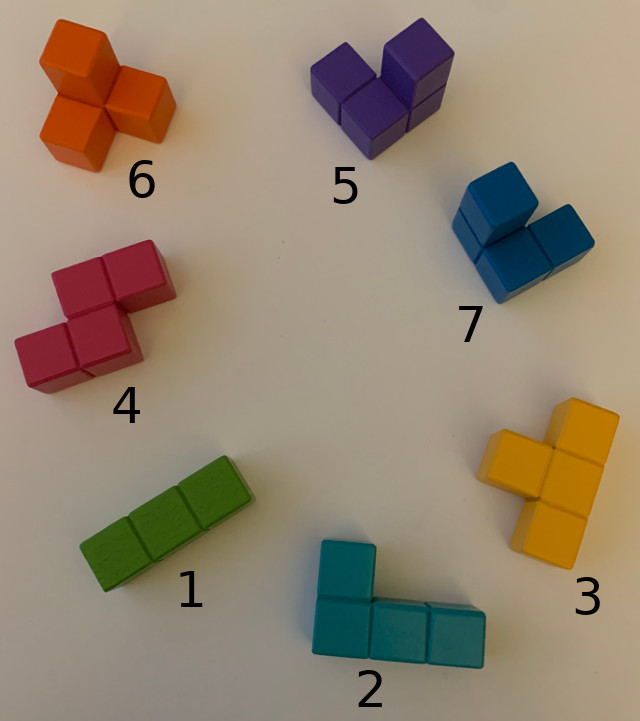This puzzle is known commercially as Cubissimo, at least in France.

All the pieces are identical to puzzle 3 except the piece 1. The goal is the same. Make a big 3x3x3 cube with the 7 pieces.

Let me show you puzzle 3 and puzzle 4 side by side for easy comparison.

Pieces 5 and 7 are not at the same position in the picture. You also have to rotate them in your head to check that they are indeed the same in both puzzles. (A bit of 3D gymnastics in your head is never a bad thing, is it?)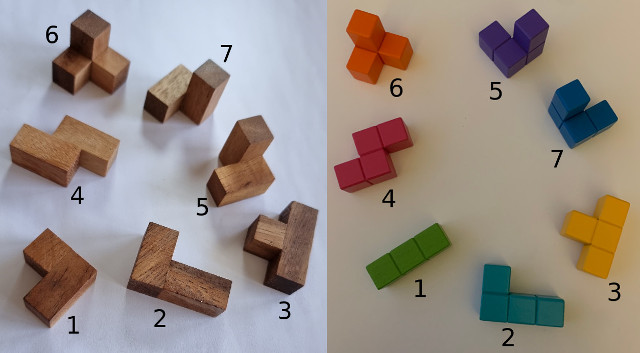The only difference is piece 1. In puzzle 3 it has an L shape. In puzzle 4 the 3 small cubes are aligned. Now the question is: which puzzle has more solutions? I bet on puzzle 3. (Writing this text, I know the answer, but I did the bet before.) Why 3? Because the L shape has more positions in the big 3x3x3 cube. (I need to prove that!) So it seems intuitive to think we'll get more solutions using it.

But let's check.

```/tmp/puzzle> tar xf cube_puzzle_4.tar.gz
/tmp/puzzle> cd cube_puzzle_4
/tmp/puzzle/cube_puzzle_4> make
gcc -g -Wall -c -o main.o main.c
gcc -g -Wall -c -o puzzle.o puzzle.c
gcc -g -Wall -o solve main.o puzzle.o
/tmp/puzzle/cube_puzzle_4> time ./solve |grep -i solution > solution_4.txt

real    10m40.060s
user    10m59.402s
sys     0m46.206s
/tmp/puzzle/cube_puzzle_4> grep solution solution_4.txt |wc -l
6624
/tmp/puzzle/cube_puzzle_4> grep solution solution_4.txt |sort|uniq|wc -l
4277
/tmp/puzzle/cube_puzzle_4> \grep solution solution_4.txt |../puzzle3_reformat_solution |sort|uniq|wc -l
276
```

We get 276 solutions. We have the same behavior with puzzle 4 than we had with puzzle 3. We have a total of 6624 solutions. Sorting them we get 4277 when we expect 6624/24 = 276. This is here also due to the inner rotations of the pieces that are not preserved when getting the canonical position of a solution. Finally the pieces can be in one of its inner rotations. Using the alternative representation of a solution given by puzzle3_reformat_solution we indeed reach 276 solutions as expected.

So we good!

And indeed, we have less solutions. And a faster running time. 10 minutes instead of 43.

Now, another question: can we design 7 pieces that would give only one solution? (I bet no. But I won't prove it!)

## Crisis moment

I just found the Geometrikum where we see puzzle 3. It is actually called Soma cube and is said to have been "invented in 1936 by Piet Hein during a lecture on quantum mechanics conducted by Werner Heisenberg". (Maybe not true. For one it does not really make sense. I mean, you're supposed to listen to your teacher. Second, french wikipedia article about the Soma cube says there is a patent from 1933 by Hein describing the puzzle, which is several years before the lecture.)

Both sources say that there are... 240 solutions! not 480 as I get.

This is bad. Where is the x2 factor that I miss? Time to think...

The Geometrikum says "There are 240 distinct solutions of the Soma cube puzzle, except for when the cube is rotated or flipped." Rotation, I got it. There are 24 of them. But flipped? What does that mean? Is it a general thing or specific to the shape of the pieces of the Soma cube? What about puzzle 4? Is there also a x2 factor I miss? Or is it even worse than x2? x6 maybe?

Wikipedia gives a link to a book by world famous Martin Gardner, The Second Scientific American Book of Mathematical Puzzles & Diversions published in 1961 that says "More than 230 essentially different solutions (not counting rotations and reflections) have been tabulated by Richard K. Guy of the University of Malaya, in Singapore, but the exact number of such solutions has not yet been determined."

The important bit is here is reflections.

So it is about reflections, like in a mirror. So I guess it has to do with pieces 5 and 7 which are a mirror version of each other. That may explain the x2 thing.

Let's check that.

Let's take the 480 solutions, compute their symmetry, and check that we arrive to another solution. And check that if solution i arrives to solution j then solution j reciprocally arrives to solution i.

Any symmetry should work, let's try the symmetries with x, y, and z axis.

```/tmp/puzzle> gcc -Wall -o check_symmetry check_symmetry.c
/tmp/puzzle> grep solution cube_puzzle_3/solution_3_good.txt| ./puzzle3_reformat_solution| sort|uniq| ./check_symmetry
x
y
what? no symmetry found for solution 0?
```

What? Symmetry with x axis works. Symmetry with y does not! (And neither does the one with z axis, comment the code for the y axis case and you will see.)

What is going on?

Time to think a bit.

It didn't take long!

I forgot to normalize the symmetry. We need to go to the canonical position of the symmetry. It is not needed for the x axis case because the small cubes used for the canonical position go to (0,0,0), (0,0,1), and (0, 1, 1). So the canonical position of the symmetry is good, no need to rotate. For y and z axis we need to rotate.

And we have a problem. Using the files we produced so far we can't check. Why? Because the result of running puzzle3_reformat_solution removes the information of which small cube of a piece is where. We only keep the information that "piece n occupies those positions in the 3x3X3 big cube".

We need to keep the information of solution_3_good.txt but order using the information output by puzzle3_reformat_solution.

Fortunately both programs 'sort' and 'uniq' have options.

Let's output a solution as:

```2 1 1 2 1 [...] 1 2 2 1 1 2 +640000 4980000 1009040 2012010 609 826 24180
```

First the solution more or less in the original format (without parentheses) as 27*3 numbers occupying exactly 162 characters so that we can use an option of 'uniq' to skip them. Then a + sign as a field separator for 'sort'. Then the solution printed in the alternative format.

A few lines of code later (I should prove in a way or another that the programs are correct) here is what we get.

```/tmp/puzzle> gcc -Wall -o puzzle3_reformat_solution_full puzzle3_reformat_solution_full.c
/tmp/puzzle> gcc -Wall -o check_symmetry_good check_symmetry_good.c
/tmp/puzzle> grep solution cube_puzzle_3/solution_3_good.txt |
./puzzle3_reformat_solution_full |sort -t + -k 2|uniq -s 162|
./check_symmetry_good | wc -l
x
y
z
240
/tmp/puzzle> grep solution cube_puzzle_3/solution_3_good.txt |
./puzzle3_reformat_solution_full |sort -t + -k 2|uniq -s 162|
./check_symmetry_good > solution_3_240.txt
x
y
z
```

(I cheated a bit, I reformatted the last two command lines, all should be on just one line for each of them, not three.)

We good! All the symmetries work. (We could do more symmetries by the way with various planes, exercise left to the reader.) And we output the 240 unique solutions.

I'm not totally convinced that there are 240 and not 480 solutions. This symmetry thing swaps two pieces. So it's not really the same solution. On the other hand, once you find a solution, the symmetric solution is trivially there, so why not count both as only one? (Also I did not think of it by myself and was chocked when I saw those other web pages claiming 240 and not 480 solutions. My ego is not happy.) (I will survive, right?) (Right?)

125 cube puzzle.

[TODO]

## Fifth Puzzle

The fifth Puzzle is different. The goal is a 6x6x6 cube. We have 54 identical small pieces, each made of 4 small cubes, organized in a T shape.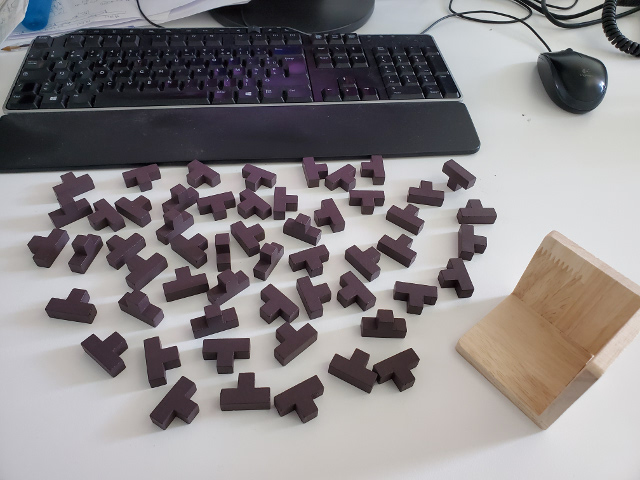Since it's so different, having only one piece, we won't use the solver of previous puzzles. That would not work well. (Can I prove it? Well, it's super intuitive, so, well, no.)

Instead of trying all positions for piece 1, then all positions for piece 2, and so on until we were able to put all the pieces (which is basically what the solver for previous puzzles does), we will work with the positions in the big 6x6x6 cube. For each position we will check all possible ways to put a piece covering that position and then go to the next. When all positions are covered by a piece, boom, solution.

How many solutions can we expect? How long to find them all? How long to find at least one?

We will answer these questions after writing some code.

I don't remember where I get this puzzle from. Someone must have given it to me. Who? I don't remember! What a horrible feeling...

When I opened it, it was solved. When disassembling it I think it was organized in three blocks of 6x6x2 small cubes. We can use this fact (if correct) to speed up the search of a solution, whatever solution.

For counting all the solutions, well, we can't.

We should also take care of rotations and symmetries. And we will say that a solution where pieces x and y exchange their positions is the same as the original one (we don't distinguish between the pieces since they all share the same shape).

Enough blabla, let's write some code to solve the 6x6x2 sub-problem.

First thing to do is to find all the possible ways to put a piece to cover a given position in the 6x6x6 cube. A piece covers a position in the big cube if any of its small cubes is at the given position. Because of the T shape of a piece, we only need to check three small cubes. The fourth, end of the horizontal top of the T, is the same as the other end, so no need to check it, we would reach a solution already found.

Since I'm too lazy to get this information by myself, I wrote a program to do it for me.

Was the process longer or shorter in the end? I don't know. I'm so used to write code that I have more trust in my program than in my thinking to find all the possible ways to put a piece to cover a given position in the 6x6x6 cube. (Hey it's a 6x6x6 cube! it's a beasty cube! funny.)

```/tmp/puzzle> cd cube_puzzle_5
/tmp/puzzle/cube_puzzle_5> gcc -Wall -o piece_positions piece_positions.c
/tmp/puzzle/cube_puzzle_5> ./piece_positions |sort|uniq|wc -l
48
/tmp/puzzle/cube_puzzle_5> ./piece_positions |sort|uniq
{ {-1, -1, 0, }, {-1, 0, 0, }, {-1, 1, 0, }, {0, 0, 0, },},
{ {-1, -1, 0, }, {0, -1, 0, }, {0, 0, 0, }, {1, -1, 0, },},
{ {-1, -1, 0, }, {0, -2, 0, }, {0, -1, 0, }, {0, 0, 0, },},
{ {-1, 0, -1, }, {-1, 0, 0, }, {-1, 0, 1, }, {0, 0, 0, },},
{ {-1, 0, -1, }, {0, 0, -1, }, {0, 0, 0, }, {1, 0, -1, },},
{ {-1, 0, -1, }, {0, 0, -2, }, {0, 0, -1, }, {0, 0, 0, },},
{ {-1, 0, 0, }, {0, -1, 0, }, {0, 0, 0, }, {0, 1, 0, },},
{ {-1, 0, 0, }, {0, -1, 0, }, {0, 0, 0, }, {1, 0, 0, },},
{ {-1, 0, 0, }, {0, 0, -1, }, {0, 0, 0, }, {0, 0, 1, },},
{ {-1, 0, 0, }, {0, 0, -1, }, {0, 0, 0, }, {1, 0, 0, },},
{ {-1, 0, 0, }, {0, 0, 0, }, {0, 0, 1, }, {1, 0, 0, },},
{ {-1, 0, 0, }, {0, 0, 0, }, {0, 1, 0, }, {1, 0, 0, },},
{ {-1, 0, 1, }, {0, 0, 0, }, {0, 0, 1, }, {0, 0, 2, },},
{ {-1, 0, 1, }, {0, 0, 0, }, {0, 0, 1, }, {1, 0, 1, },},
{ {-1, 1, 0, }, {0, 0, 0, }, {0, 1, 0, }, {0, 2, 0, },},
{ {-1, 1, 0, }, {0, 0, 0, }, {0, 1, 0, }, {1, 1, 0, },},
{ {-2, 0, 0, }, {-1, -1, 0, }, {-1, 0, 0, }, {0, 0, 0, },},
{ {-2, 0, 0, }, {-1, 0, -1, }, {-1, 0, 0, }, {0, 0, 0, },},
{ {-2, 0, 0, }, {-1, 0, 0, }, {-1, 0, 1, }, {0, 0, 0, },},
{ {-2, 0, 0, }, {-1, 0, 0, }, {-1, 1, 0, }, {0, 0, 0, },},
{ {0, -1, -1, }, {0, -1, 0, }, {0, -1, 1, }, {0, 0, 0, },},
{ {0, -1, -1, }, {0, 0, -1, }, {0, 0, 0, }, {0, 1, -1, },},
{ {0, -1, -1, }, {0, 0, -2, }, {0, 0, -1, }, {0, 0, 0, },},
{ {0, -1, 0, }, {0, 0, -1, }, {0, 0, 0, }, {0, 0, 1, },},
{ {0, -1, 0, }, {0, 0, -1, }, {0, 0, 0, }, {0, 1, 0, },},
{ {0, -1, 0, }, {0, 0, 0, }, {0, 0, 1, }, {0, 1, 0, },},
{ {0, -1, 0, }, {0, 0, 0, }, {0, 1, 0, }, {1, 0, 0, },},
{ {0, -1, 1, }, {0, 0, 0, }, {0, 0, 1, }, {0, 0, 2, },},
{ {0, -1, 1, }, {0, 0, 0, }, {0, 0, 1, }, {0, 1, 1, },},
{ {0, -2, 0, }, {0, -1, -1, }, {0, -1, 0, }, {0, 0, 0, },},
{ {0, -2, 0, }, {0, -1, 0, }, {0, -1, 1, }, {0, 0, 0, },},
{ {0, -2, 0, }, {0, -1, 0, }, {0, 0, 0, }, {1, -1, 0, },},
{ {0, 0, -1, }, {0, 0, 0, }, {0, 0, 1, }, {0, 1, 0, },},
{ {0, 0, -1, }, {0, 0, 0, }, {0, 0, 1, }, {1, 0, 0, },},
{ {0, 0, -2, }, {0, 0, -1, }, {0, 0, 0, }, {0, 1, -1, },},
{ {0, 0, -2, }, {0, 0, -1, }, {0, 0, 0, }, {1, 0, -1, },},
{ {0, 0, 0, }, {0, 0, 1, }, {0, 0, 2, }, {0, 1, 1, },},
{ {0, 0, 0, }, {0, 0, 1, }, {0, 0, 2, }, {1, 0, 1, },},
{ {0, 0, 0, }, {0, 1, -1, }, {0, 1, 0, }, {0, 1, 1, },},
{ {0, 0, 0, }, {0, 1, -1, }, {0, 1, 0, }, {0, 2, 0, },},
{ {0, 0, 0, }, {0, 1, 0, }, {0, 1, 1, }, {0, 2, 0, },},
{ {0, 0, 0, }, {0, 1, 0, }, {0, 2, 0, }, {1, 1, 0, },},
{ {0, 0, 0, }, {1, -1, 0, }, {1, 0, 0, }, {1, 1, 0, },},
{ {0, 0, 0, }, {1, -1, 0, }, {1, 0, 0, }, {2, 0, 0, },},
{ {0, 0, 0, }, {1, 0, -1, }, {1, 0, 0, }, {1, 0, 1, },},
{ {0, 0, 0, }, {1, 0, -1, }, {1, 0, 0, }, {2, 0, 0, },},
{ {0, 0, 0, }, {1, 0, 0, }, {1, 0, 1, }, {2, 0, 0, },},
{ {0, 0, 0, }, {1, 0, 0, }, {1, 1, 0, }, {2, 0, 0, },},
```

We get 48 ways to put a piece to cover a position (provided there are no border and no other piece around) (and also we have negative values in there, but it's for later use). After a bit of thinking, I feel confident. We indeed have 48 (unique) ways, no more, no less.

Now we can use this data and write a solver.

First, let's check if we can solve a 6x6x2 (note the last 2, not 6) shape (I can't write "cube", 6x6x2 is not a cube, even if in my head it is).

```/tmp/puzzle> cd cube_puzzle_5
/tmp/puzzle/cube_puzzle_5> gcc -Wall -o solve662 solve662.c
solve662.c: In function 'p':
solve662.c:109:14: warning: zero-length gnu_printf format string [-Wformat-zero-length]
printf("");
^~
solve662.c:111:12: warning: zero-length gnu_printf format string [-Wformat-zero-length]
printf("");
^~
/tmp/puzzle/cube_puzzle_5> time ./solve662 |wc -l
4896

real    0m2.353s
user    0m2.355s
sys     0m0.000s
/tmp/puzzle/cube_puzzle_5> time ./solve662 |sort|uniq|wc -l
136

real    0m2.356s
user    0m2.347s
sys     0m0.016s
```

First of all, does it work? Yes! See this picture. I just took one solution (I don't know which one, any is good) to do the first two 6x6x2 blocks and another one (to check) for the third 6x6x2 block, and there we go!

(I had to edit the program to print a solution on several lines, see the function p(), put \n at the end of all the printf() that don't have it already (except one, which one? exercise for the reader) and you get something you can read and use to solve the puzzle, I mean assemble.)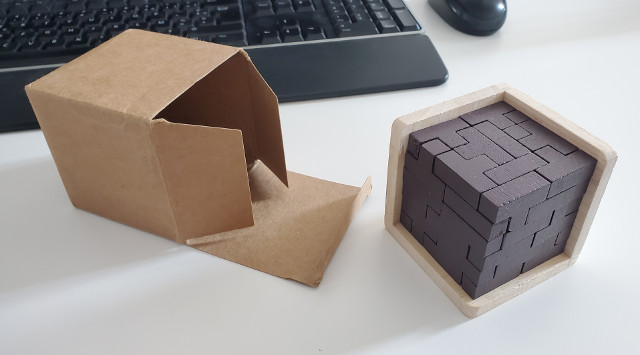But we have a problem.

We get 4896 solutions but using sort|uniq we get 136 unique solutions. That does not make sense! The program should not output a solution more than once!

I need to think...

The running time is quiet fast, only 2 seconds.

At first the program was full of bugs. Bad copy/paste where I forget to change x by y and z, then I didn't use nx, ny, and nz in go() so x, y, and z were directly modified leading to some crazy things, then I forgot to handle the case if (busy[x][y][z]) in the functio go(), then I put it but at the wrong place! So many bugs for a program of less than 200 lines, that's crazy.

And it was super slow, which I expected, so I let it run for a while. Then I thought that there might be bugs in there, so I tried a 3x3x3 version. No solution should pop up, it's impossible because we have 27 positions and a piece has 4 small cubes, so you can't find n pieces to cover 27 positions. But the program found some!

Insane.

And funny.

And scary.

But the picture proves that the program works in the end. Unless you don't believe me when I say the program found the solution and not my brain. But that's another story.

Why so many solutions... and why not unique!?

## After some classical debugging

I was hoping my brain would find the bug while sleeping. But no. So I reverted to some classical debugging.

First question I had was: is piece_positions.c correct? Do we really have 48 ways to put a piece to cover a position? I visualized it hard in my head and I was almost sure that yes. I nevertheless played a bit with piece_positions.c, putting some printf() here and there to print a solution in different ways, but nothing came out it.

I'm still not 100% sure that piece_positions.c is correct though. I don't have a proof. But thinking and visualizing stuff in my head I don't see how we could have more (or less) than 48 ways to blabla. So, well, the bug was not there.

Then I did the same thing with solve662.c and after an hour or two of printing stuff and wondering what was going on, I finally found that I messed up with the end condition of the recursion of function go(). You can see in the code:

```  if (z == 2) { p(); /*exit(1);*/ }
```

At first I was only interested in one solution, so I put this exit(1).

Then I wanted all the solution, so, well, easy, remove the exit(1). Except! now the recursion does not terminate properly. If z == 2 it means we have a solution and we have to print it and then we have to return! not execute the code below the if().

Anything can happen when running it with z == 2. By luck (really?) the program did not crash and just printed the same solution 36 times. (36 is 6x6, it's the range of x and y dimensions of the 6x6x2 'cube'.) (Not that it means something. It could have happened literally anything.)

I didn't save all the printf() I put, but for reference, here is solve662_analyze.c, its last version, let's say.

Running it piped to the command less, and looking for "Q \[0 0 0 2] \[1 0 0 35] \[2 0 0 39] \[3 0 0 6] \[3 1 0 2] \[4 1 0 3] \[5 1 0 21] \[1 2 0 39] \[2 3 0 35] \[5 3 0 2] \[0 4 0 21] \[2 4 0 7] \[0 5 0 6] \[4 5 0 35] \[3 0 1 40] \[1 1 1 40] \[4 2 1 3] \[1 4 1 7]" (why looking for this? because it's a solution that was printed several times by the program, so it was a good candidate for further analysis) (without quotes) (yes it's big, sorry, download the html for better view if you need) in less, we can see this (I cut long lines, we don't care about their content):

```[...]
go 4 5 1 19
busy[4 5 1]
go 5 5 1 19
busy[5 5 1]
go 0 0 2 19
------------------ 01 02 03 04 04 04 01 03 [...] 11 13 18 14 14 14
Q [0 0 0 2] [1 0 0 35] [2 0 0 39] [3 0 0 6] [...] [4 2 1 3] [1 4 1 7]
busy[0 0 2]
go 1 0 2 19
------------------ 01 02 03 04 04 04 01 03 [...] 11 13 18 14 14 14
Q [0 0 0 2] [1 0 0 35] [2 0 0 39] [3 0 0 6] [...]  [4 2 1 3] [1 4 1 7]
busy[1 0 2]
go 2 0 2 19
------------------ 01 02 03 04 04 04 01 03 [...] 11 13 18 14 14 14
Q [0 0 0 2] [1 0 0 35] [2 0 0 39] [3 0 0 6] [...] [4 2 1 3] [1 4 1 7]
busy[2 0 2]
go 3 0 2 19
------------------ 01 02 03 04 04 04 01 03 [...] 11 13 18 14 14 14
Q [0 0 0 2] [1 0 0 35] [2 0 0 39] [3 0 0 6] [...] [4 2 1 3] [1 4 1 7]
busy[3 0 2]
go 4 0 2 19
[...]
```

See, we call go(0, 0, 2, 19) (which is fine), but then we also call go(1, 0, 2, 19), go(2, 0, 2, 19), go(3, 0, 2, 19), and go(4, 0, 2, 19), which should not happen. I saw this log, I knew it was wrong, I found the bug, I'm happy.

With a return after p() the program outputs only 136 solutions. Verification left to the reader (come on, it's easy, just add return, recompile, run without sort|uniq, then run with sort|uniq and compare) (you can pipe to md5sum for example to check that it's identical, I do it all the time) (ah, but you should run with sort for the first run, to get the lines in the same order as for the sort|uniq case) (you can also simply count the lines with piping with wc -l, you will get 136).

Now another question pops up. Why 136 solutions? I mean, okay, why not. But the problem is that counting rotations and symmetries of the 6x6x2 'cube' we should have 16 of them (I think, but I guess I'm wrong). Four rotations for the cube with one 6x6 side up. Then four others for the other side up. We get 8. But then there is a symmetry (mirror symmetry) like for the 3x3x3 cube of the previous puzzles, no? That would give 2x8 identical solutions. But 136 is not multiple of 16. It is multiple of 8. 136 = 17x8. So we really get 17 unique solutions (taking into account rotations) (but not the mirror symmetry, why?).

Maybe because the piece is unique and the mirror version of it is... it? So we would simply reach another solution in the 17 unique ones?

That thinking needs some analysis and some code.

## Time flies away and never comes back

...After some programming, I find 10 unique solutions. See by yourself.

```/tmp/cube_puzzle> cd puzzle_5
/tmp/cube_puzzle/puzzle_5> gcc -Wall 662count.c -o 662count
662count.c: In function ‘p’:
662count.c:141:14: warning: zero-length gnu_printf format string [-Wformat-zero-length]
141 |       printf("");
|              ^~
662count.c:143:12: warning: zero-length gnu_printf format string [-Wformat-zero-length]
143 |     printf("");
|            ^~
/tmp/cube_puzzle/puzzle_5> ./662count < sol662.txt |grep \\------|wc -l
10
```

I don't really trust my code.

In the function check_solution() the first for() loop needs to handle only three cases (we don't want to test the solution itself, obviously it will be equal to itself) but then for the following for() loops we have to deal with four, not three, and at first I just copied/pasted the first loop and forgot to think again about the limits of the loop and kept 3, not 4. That gave me 31 solutions, not 10.

(Oh, sol662.txt is the output of "./solve662 |sort|uniq" without the leading ------------------, you can generate it easily by yourself, don't be so lazy! It should be 136 lines long and "md5sum sol662.txt" says b99db1d5b8dafcd96d851f0e44c16b74.)

Since I don't trust my code, I need to be a bit more convinced. How? For each of the 136 solutions I will generate all the rotations/symmetries and see how many unique positions I get. We have 10 solutions from 136, so I bet on average each of the 136 solutions should have... wait I don't know, but less than 16 unique positions. (I was going to say 13.6 but that's a wrong thinking, I think.) (I think super badly today.) (By the way this webpage has been written over many many days.)

Let's write some code to check. Let's hope I don't put too much bugs in there...

## Bugs bugs bugs...

I wrote some code. uniq662.bad.c (run as ./uniq662.bad < sol662.txt) is the result. As the name implies, the code is bad. What is it supposed to do? For each of the 136 solutions it gets all the rotations/symmetries and stores the unique versions into an array and then prints the size of the array. We get 6 or 7 for all the solutions. I don't know if this is correct or not but after reading the function feed_sol() I realized I did not generate all the rotations.

We have this kind of loops.

```  rotate2(s, s3);
normalize(s3, s2);
for (i = 0; i < 4; i++) {
rotate1(s2, s3);
normalize(s3, sn);
if (!in_sol(sn)) put(sn);
}
```

The < 4 is good but then we do: s3 = rotate1(s2); sn = normalize(s3); and we repeat. That means at each loop we compute the same s3 and the same sn. So we check the same rotation over and over.

So I wrote uniq662.c, fixing all those bugs (I hope, not sure actually). And this time it does not print 6 or 7 but 16 or 8. Which looks... better? more normal? Actually I don't know.

The bug does not seem to be present in 662count.c. So I guess we really have only 10 unique solutions. I'm not super confident though.

The function feed_sol() is super low level. The use of s2, s3, sn, and sx is like using registers when programming in assembly language. You need to be careful to know what value is where and how to use it and store it in another variable and which one. It really reminds me my youth when I was doing assembly language like a barbarian (but I wrote a text editor and a debugger in assembly language and many small programs). A friend of mine wrote a 3D game in assembly language at the time (Hulud, if you read this!) and his code was so much cleaner. Anyway, just to say that switching from assembly to C frees you from those low level details of register allocation. The compiler deals with that for you. So when you write a C function where you need to be careful again with your variables as if they were registers of the computer when writing some assembly language code, then you know you are doing something wrong, and you will most certainly write bad code full of bugs. At the least you should have a very low trust in this code and try to rewrite it in a more elegant high level way.

Maybe I should rewrite 662count.c then?

Maybe.

How to be sure I get only 10 solutions? Difficult question.